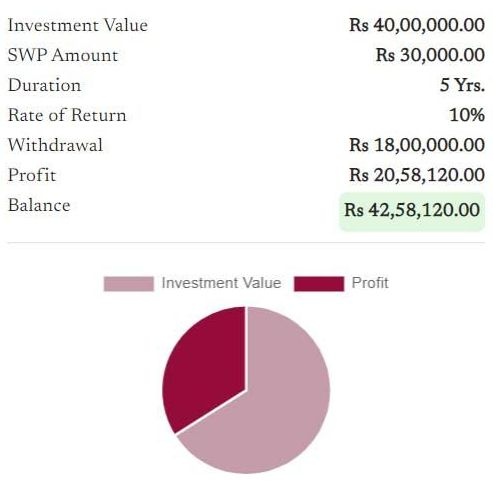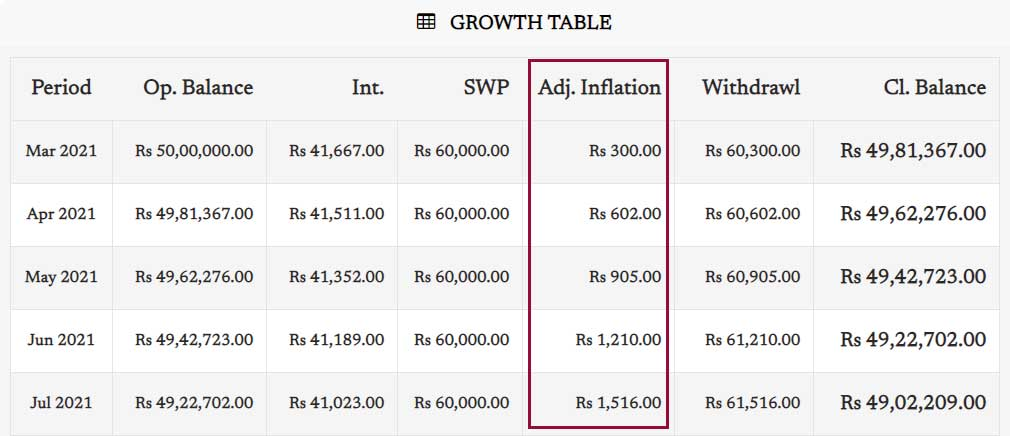SWP Calculator | Systematic Withdrawal Plan Calculator With Inflation

# SWP Calculator | Systematic Withdrawal Plan Calculator With Inflation

SWP or Systematic Withdrawal Plan Calculator lets you estimate your periodic withdrawals over an investment.

Systematic Withdrawal Plan (SWP) Calculator enables you to measure how much you can earn on an interval (monthly, quarterly, or annually) from a lump sum investment over a mutual fund. Let's take as an example, you have a lump sum amount of around 50 lakh, and you expect to generate a regular income of 60 thousand by the amount per month. In this situation, SWP can be beneficial for you. You can invest your funds in any mutual fund and avail of a SWP (Systematic Withdrawal Plan). A fixed amount (As per your choice e.g.... 60k/ month) will get credited into your savings account periodically until the period you have chosen, or the balance of the fund gets lower than the monthly income. During the phase of periodical withdrawals, your funds will also grow as per the fund's performance. Here is an example:

 Investment Rs. 50,00,000.00 (50 Lakh) SWP Amount Rs. 60,000.00 / Month Duration 10 Yrs. Rate of Return 8% (CAGR) Total Withdrawal Rs. 72,00,000.00 (72 Lakh) Total Profit Rs. 23,21,439.00 (23 Lakh) Balance (After 10 Years) Rs. 1,21,439.00 (1.21 Lakh)

## How to use the SWP Calculator?

Using the SWP Calculator is very easy. All you need to fill in the following inputs and click on the 'Calculate Now' button to get the result:

• Initial Investment Amount (Rs.): The lumpsum amount you want to invest in a mutual fund.
• SWP/ Monthly Withdrawal Amount (Rs.): The amount you want to withdraw monthly.
• Duration: How long do you want to continue the SWP
• Expected Rate of Return (%): Your expected return (in percentage) from the mutual fund you are going to invest
• Rate of Inflation (%): This is an optional input. If you want an inflation-adjusted return calculation, you can input the rate of inflation (in percentage).

The calculation result contains estimated profit, fund balance after the fund life, an investment summary chart, and a growth table. The growth table represents the balance sheet of the SWP.## How to measure inflation with the SWP Calculator?

Measuring inflation over investments is very important. Inflation is the factor that reduces the value of the rupee or any currency over time. For example, the value of 100 rupees will depreciate to 92 rupees next year if the inflation is around 7% per annum. So, taking into consideration inflation while calculating the return on investments is a good financial practice. With Finlive's SWP Calculator, you can consider inflation during the calculation. All you need to fill in the 'Rate of Inflation (%)' at the time of calculating. Eg. If you think the average rate of inflation will be around 6%, just input 6 in the 'Rate of Inflation (%) field and click on the 'Calculate Now' button. In the 'Growth Table,' you can find the adjusted inflation in each row.## Benefits of Using SWP Calculator

• It's FAST! The SWP Calculator can save a lot of time and effort in calculating the complex SWP manually.
• It's easy to use. The calculator is designed in such a way that any person can utilize it.
• You can estimate your approx monthly income by an SWP.
• SWP Calculator lets us measure inflation-adjusted return.
• The best thing about the SWP Calculator is it's FREE.

## Who can use the SWP Calculator?

Finlive's SWP Calculator is FREE to use a financial calculator. Anyone who wants to estimate his/ her earnings from a Systematic Withdrawal Plan (SWP) can use the SWP Calculator. It's easy to use and less time-consuming. Calculating SWP manually can take a lot of time and effort. Here, you only need to input a few details and get the result within a second.

Suggested Calculators